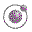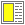Name binding, Recursion, Iteration, and Continuations - slide 41 : 42

Practical example: Length of an improper list
The length of an improper list is undefined

We chose to return the symbol improper-list if list-length encounters an improper list

This example is similar to the catch and throw example shown earlier in this section

```(define (list-length l)
(call-with-current-continuation
(lambda (do-exit)
(letrec ((list-length1
(lambda (l)
(cond ((null? l) 0)
((pair? l) (+ 1 (list-length1 (cdr l))))
(else (do-exit 'improper-list))))))
(list-length1 l)))  ))```